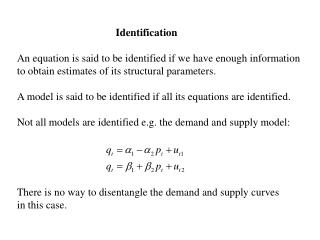DownloadDownload PresentationIdentification

Identification

Télécharger la présentationIdentification

- - - - - - - - - - - - - - - - - - - - - - - - - - - E N D - - - - - - - - - - - - - - - - - - - - - - - - - - -
Presentation Transcript

1. Identification An equation is said to be identified if we have enough information to obtain estimates of its structural parameters. A model is said to be identified if all its equations are identified. Not all models are identified e.g. the demand and supply model: There is no way to disentangle the demand and supply curves in this case.

2. The exogenous variables of a model help to identify its equations To identify the demand curve we need to find a variable which affects supply but not demand. Note that establishing that an equation is identified tells us that it can be estimated. It does not tell us how to estimate it.

3. The simultaneous equations model in matrix form The structural form of the SEM model can be written in the following form: where yis a Gx1 vector of endogenous variables, x is a Kx1 vector of exogenous variables and u is a Gx1 vector of random errors. B and Γ are GxG and GxK matrices of coefficients.

4. For example, we could write the demand-supply model as: If the B matrix is invertible then we can solve for the reduced form of the model as: where Π is the GxK matrix of reduced-form coefficients.

5. Relationship between the reduced form and the structural form An equation is identified if there is enough information in the reduced form to solve for the structural form. The relationship between the reduced form and structural form parameters can be written: If there are no restrictions on the structural form then it will be impossible to estimate. We can determine the number of restrictions we need as follows.

6. We can write the relationship between the structural and reduced forms as: The i’th row of the matrix A(αi) contains the structural parameters of equation i. Without restrictions there are G+K structural parameters in each equation. To solve for these we have K equations which are defined by: Since we have G+K unknowns and only K equations, it follows that we need R≥G where R is the number of restrictions.

7. Types of Restriction Almost all equations make use of a normalisation restriction i.e. the coefficient on the LHS variable is set equal to one. This reduces the number of restrictions required to R ≥ G-1. Exclusion Restrictions occur when we leave a variable out of the equation (set its coefficient equal to zero). If exclusion restrictions are the only type used, then we have R = (G-g) + (K-k) and the condition for identification becomes K-k ≥ g-1. This is the order condition for identification. (Note that g and k are the numbers of endogenous and exogenous variables included in equation i).

8. The order condition for identification If K-k = g-1 then the equation is said to be just identified. If K-k > g -1 then the equation is said to be over identified. If K-k < g-1 then the equation is said to be under identified. Note that the order condition is a necessary but not sufficient condition for identification when exclusion restrictions are the only restrictions applied. Note also that equations can be identified by other types of restriction.

9. In the following example, the demand curve is just identified In the next example, the demand curve is over identified

10. Finally, in this example, the demand curve is not identified. If an equation is just identified then we can obtain unique estimates of the structural parameters from the reduced form. This is known as the method of Indirect Least Squares.